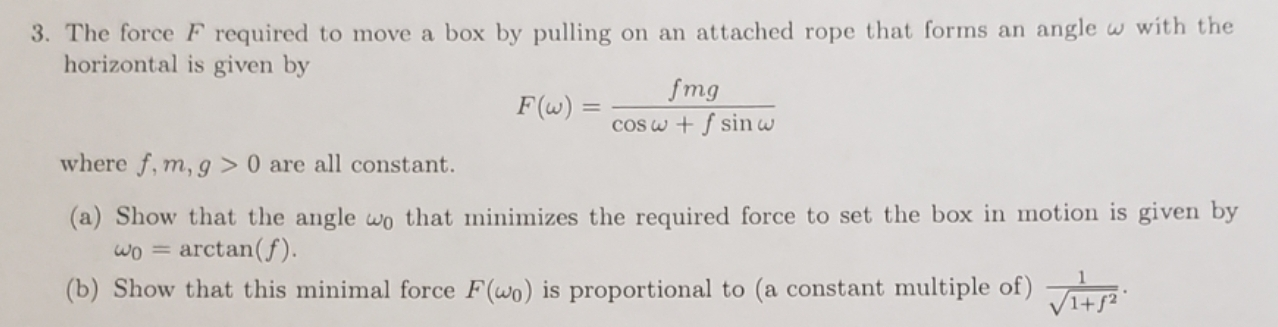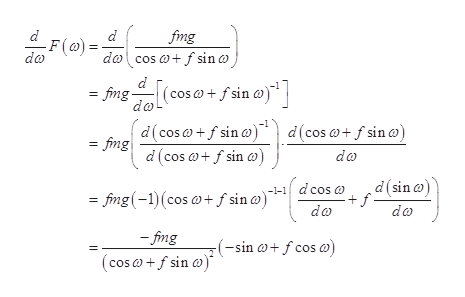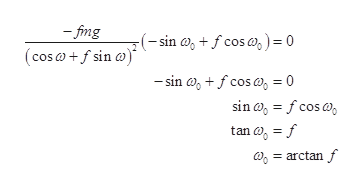# 3. The force F required to move a box by pulling on an attached rope that forms an angle w with thehorizontal is given byfmgF(w) =cOSw + f sin wwhere f, m, g > 0 are all constant.(a) Show that the angle wo that minimizes the required force to set the box in motion is given byarctan(f)(b) Show that this minimal force F(wo) is proportional to (a constant multiple of)

Question
29 viewshelp_outlineImage Transcriptionclose3. The force F required to move a box by pulling on an attached rope that forms an angle w with the horizontal is given by fmg F(w) = cOSw + f sin w where f, m, g > 0 are all constant. (a) Show that the angle wo that minimizes the required force to set the box in motion is given by arctan(f) (b) Show that this minimal force F(wo) is proportional to (a constant multiple of) fullscreen
check_circle

Step 1

a)

Force required to move the box of mass m is,

Step 2

The quantities f, m and g are constant. ω is variable.

Differentiate the above equation with respect to ω by applying chain rulehelp_outlineImage Transcriptionclosed fmg -F() = do da cosa+ f sin o d =fimg- da (cos o+ fsin @)| d(cos af sin a)d(cos f sin ) fing d(cos af sin a = da .d(sin) --1 dcos o f da - fimg(-1)(cos + f sin ) do -fmg (-sin fcos a (cos+f sin a) fullscreen
Step 3

Calculate ω for mini...help_outlineImage Transcriptionclose-fing (-sin a,fcos a,) = 0 (cos a+f sin a -sin fcosa, = 0 sin a fcos an o = f aarctanf fullscreen

### Want to see the full answer?

See Solution

#### Want to see this answer and more?

Solutions are written by subject experts who are available 24/7. Questions are typically answered within 1 hour.*

See Solution
*Response times may vary by subject and question.
Tagged in

### Physics